Processing ......FreeComputerBooks.com Links to Free Computer, Mathematics, Technical Books all over the World

Real Variables with Basic Metric Space Topology
Top Free C Programming Books 🌠 - 100% Free or Open Source!
• Title Real Variables with Basic Metric Space Topology
• Author(s) Robert B. Ash
• Publisher: Dover Publications (May 21, 2009)
• Hardcover/Paperback 224 pages
• eBook PDF Files and a single PDF (78 MB)
• Language: English
• ISBN-10/ASIN: 0486472205
• ISBN-13: 978-0486472201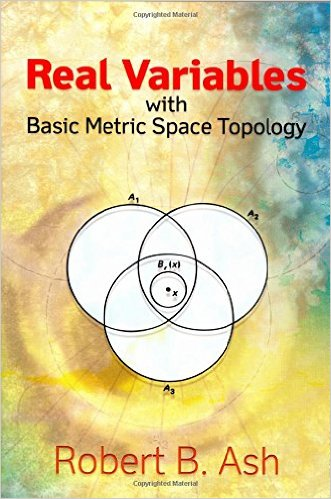Book Description

Designed for a first course in real variables, this text presents the fundamentals for more advanced mathematical work, particularly in the areas of complex variables, measure theory, differential equations, functional analysis, and probability. Geared toward advanced undergraduate and graduate students of mathematics, it is also appropriate for students of engineering, physics, and economics who seek an understanding of real analysis.

The author encourages an intuitive approach to problem solving and offers concrete examples, diagrams, and geometric or physical interpretations of results. Detailed solutions to the problems appear within the text, making this volume ideal for independent study. Topics include metric spaces, Euclidean spaces and their basic topological properties, sequences and series of real numbers, continuous functions, differentiation, Riemann-Stieltjes integration, and uniform convergence and applications.

• Robert B. Ash is Professor Emeritus of Mathematics at the University of Illinois.
Reviews, Rating, and Recommendations: Related Book Categories: Read and Download Links:Similar Books:
•Measure, Integration and Real Analysis (Sheldon Axler)

This textbook welcomes students into the fundamental theory of measure, integration, and real analysis. Focusing on an accessible approach, it lays the foundations for further study by promoting a deep understanding of key results.

•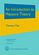An Introduction to Measure Theory (Terrence Tao)

This is a graduate text introducing the fundamentals of measure theory and integration theory, which is the foundation of modern real analysis, intended to cover a quarter or semester's worth of material for a first graduate course in real analysis.

•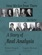How We Got from There to Here: A Story of Real Analysis

This book is an introductory real analysis textbook, presented through the lens of history. The definitions and techniques are motivated by the actual difficulties encountered by the intuitive approach and are presented in their historical context.

•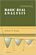Basic Real Analysis (Anthony W. Knapp)

This is a comprehensive treatment with a global view of the Real Analysis, emphasizing the connections between real analysis and other branches of mathematics. Included throughout are many examples and hundreds of problems.

•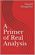A Primer of Real Analysis (Dan Sloughter)

This is a short introduction to the fundamentals of real analysis, written the text assuming the reader has the level of mathematical maturity of one who has completed the standard sequence of calculus courses.

•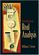Introduction to Real Analysis (William F. Trench)

Using an extremely clear and informal approach, this book introduces readers to a rigorous understanding of mathematical analysis and presents challenging math concepts as clearly as possible.

•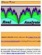Interactive Real Analysis (Bert G. Wachsmuth)

An interactive textbook for Real Analysis or Advanced Calculus in one real variable. It deals with sets, sequences, series, continuity, differentiability, integrability (Riemann and Lebesgue), topology, power series, and more.

•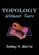Topology without Tears (Sidney A. Morris)

General topology is the branch of topology dealing with the basic set-theoretic definitions and constructions used in topology. The aim of this is to provide a thorough grouding of general topology. It offers an ideal introduction to the fundamentals of topology.

•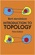Introduction to Topology (Bert Mendelson, et al.)

This concise book offers an ideal introduction to the fundamentals of topology. The book's principal aim is to provide a simple, thorough survey of elementary topics in the study of collections of objects, or sets, that possess a mathematical structure.

•Topology: A Categorical Approach (Tai-Danae Bradley, et al)

A graduate-level textbook that presents basic topology from the perspective of category theory. Many graduate students are familiar with the ideas of point-set topology and they are ready to learn something new about them.

Book Categories
 :All CategoriesTop Free BooksRecent BooksMiscellaneous BooksComputer EngineeringComputer LanguagesComputer ScienceData Science/DatabasesJava and Java EE (J2EE)Linux and UnixMathematicsMicrosoft and .NETMobile ComputingNetworking and CommunicationsSoftware EngineeringSpecial TopicsWeb Programming
Other Categories